# 一类反应扩散方程的孤立周期波和局部临界周期分支*

(1. 广西师范大学 数学与统计学院， 广西 桂林 541006；2. 广东财经大学 统计与数学学院， 广州 510320)

## 引 言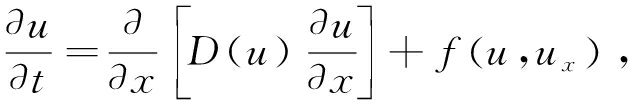(1)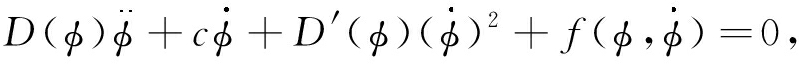(2)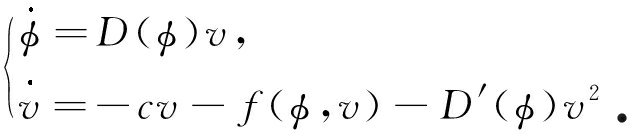(3)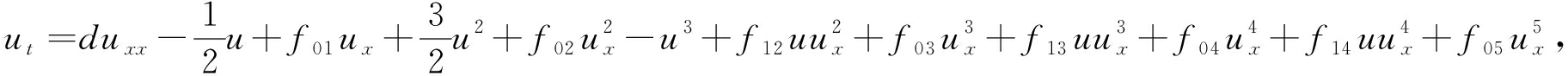(4)

## 1 预 备 知 识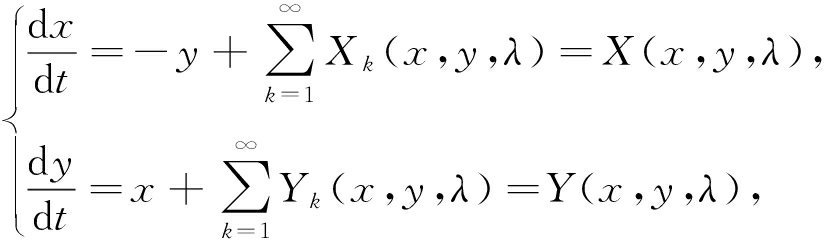(5)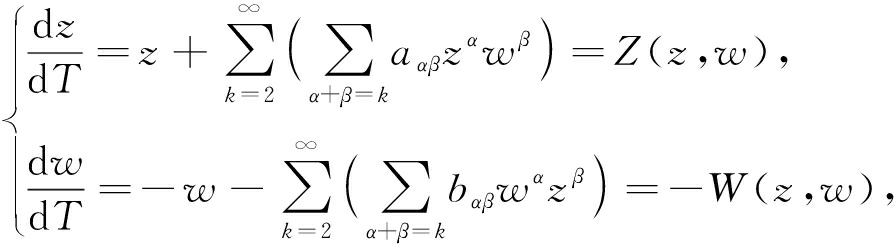(6)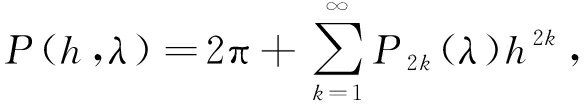(7)

P2k称为系统(5)原点的第k阶周期常数.

1) λ*∈RN的任一邻域包含有λV(f1, f2,…, fk-1)，使得fk(λ)f(λ)<0；

2) 代数簇V(f1, f2,…, fj),2≤jk-1，若λV(f1, f2,…, fj)且fj+1(λ)≠0，则λ的任一邻域W包含有σV(f1, f2,…, fj-1)，使得fj(σ)fj+1(λ)<0；

3) 若λV(f1)且f2(λ)≠0，则λ的任一开邻域包含一个σ，使得f1(σ)f2(λ)<0.

P2k=-πτk.

(8)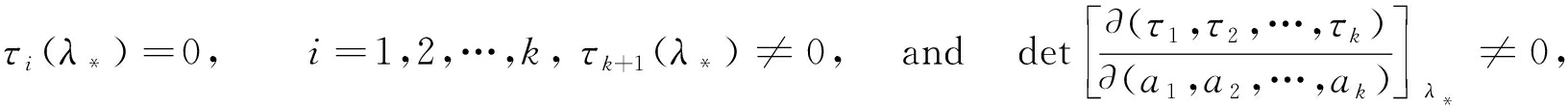(9)

## 2 奇点量、连续周期波列和SAIPW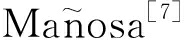受到文献的启发，提出了下述假设．这一假设构建了类型(1)非线性波方程的稳态解和对应行波系统的细焦点之间的桥梁，也是研究类型(1)非线性波方程行波解存在性的重要途径.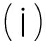f(0,0)=f(1,0)=0，f(α,0)=0，对∀α∈(0,1)；否则，f(u,0)≠0.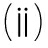f(φ,v)∈Cω(R2)，fφ(0,0)<0，fφ(α,0)>0且fφ(1,0)<0.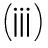D(u)∈Cω(R)，对∀u≥0，有D(u)>0.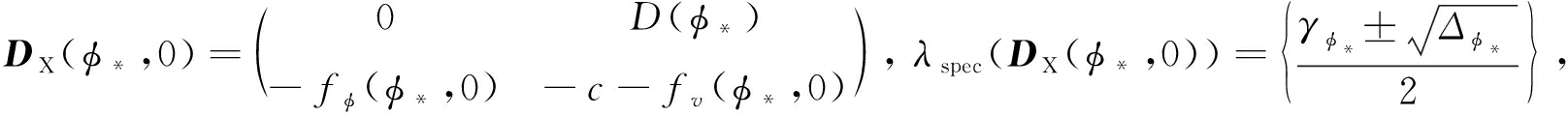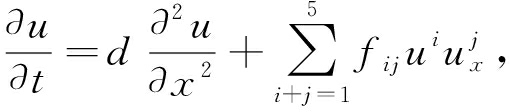(10)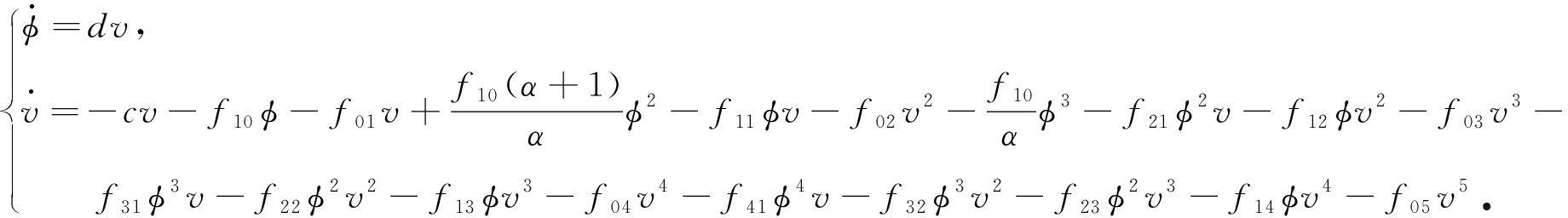(11)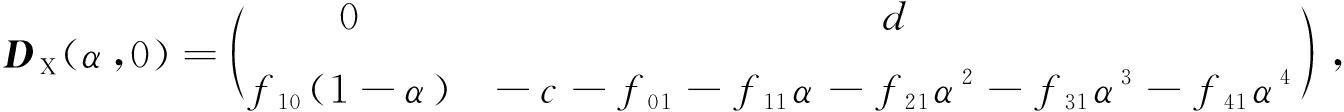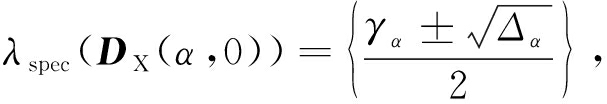γα=-c-(f01+f11α+f21α2+f31α3+f41α4),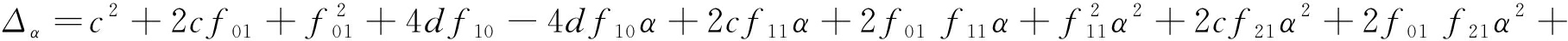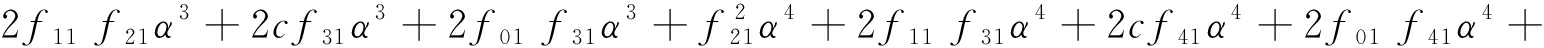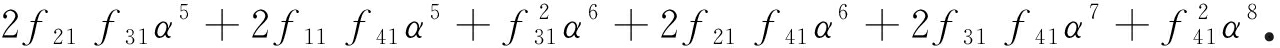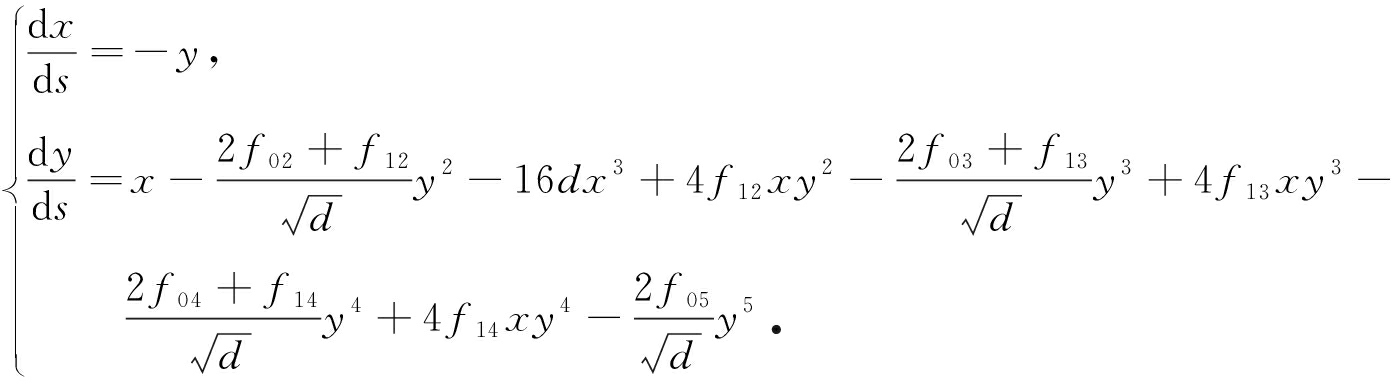(12)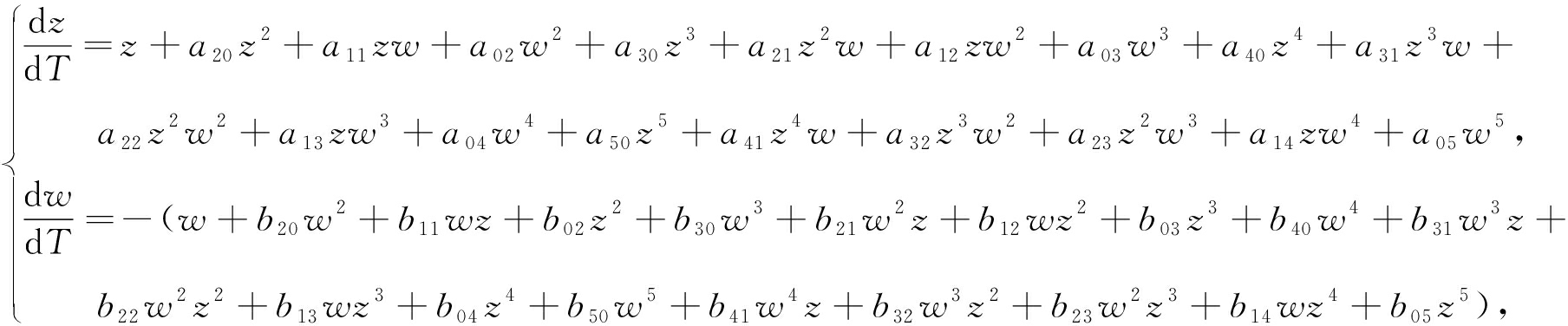(13)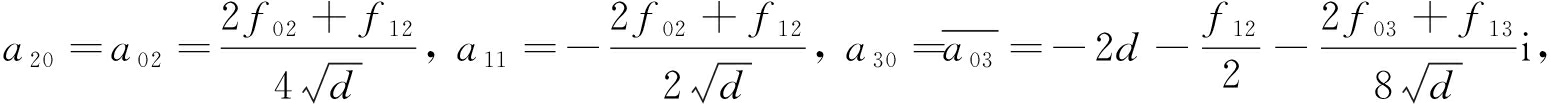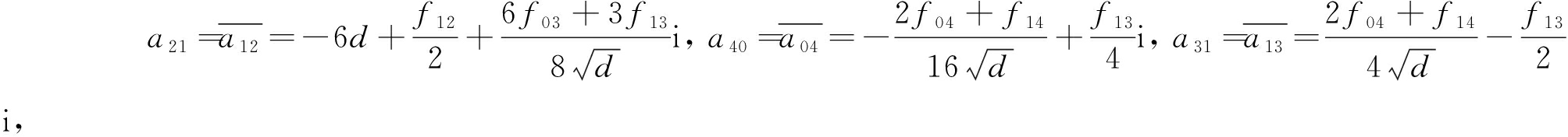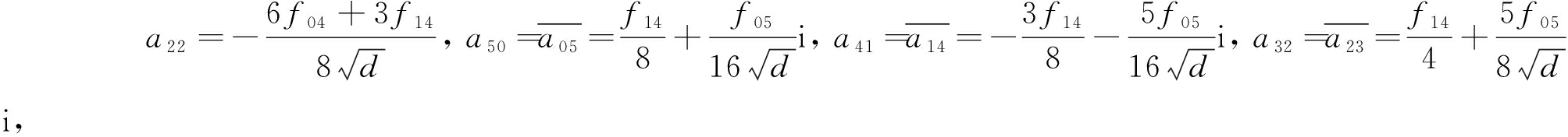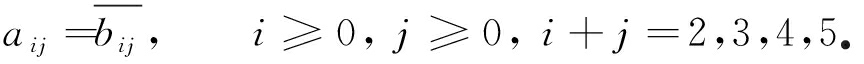K1={λ∈R9: f03=f05=f13=0};

K2={λ∈R9: 2f03+f13=0, f05=0, 2f04+f14=0, 2f02+f12=0}.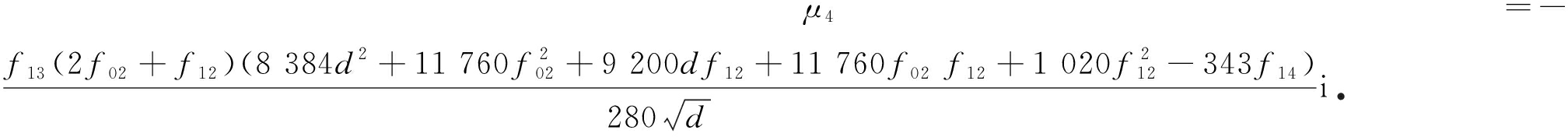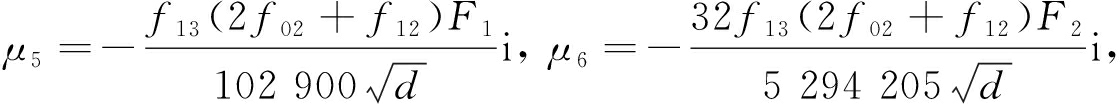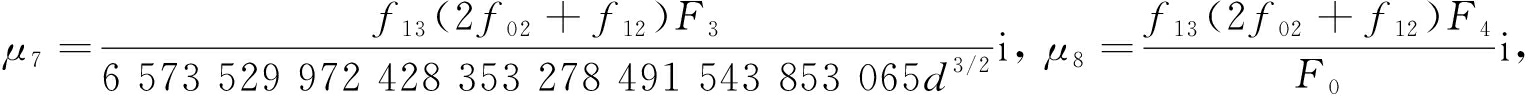F0=899 184 838 685 683 965 029 890 839 471 690 326 604 873 618 006 549

674 053 265 479 127 114 300 359 926 555 625 490 075 367 578 280d5/2,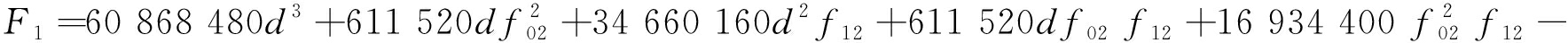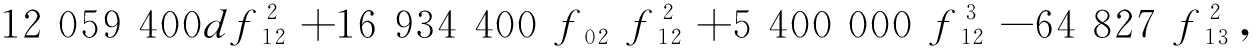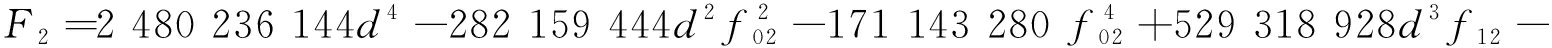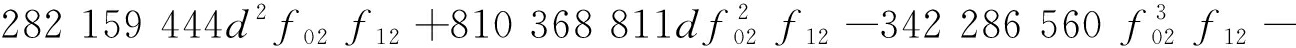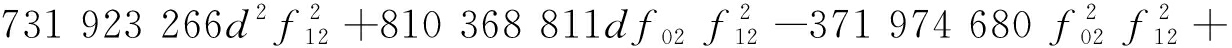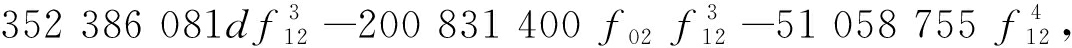F3F4也为d, f02, f12的表达式，因其表达式较复杂，故在此省略.

f13=0，则f05=f03=0且前8个奇点量全都为零．因此，我们得到条件K1.

Res(F2,F3, f02)=M1

Res(F2,F4, f02)=M2

Res(M1,M2, f12)=50 096 392 356 781 803 791 886 937 692 913 903 439 852 161 558 375 638…,

μ1=μ2=μ3=μ4=μ5=μ6=μ7=μ8=0.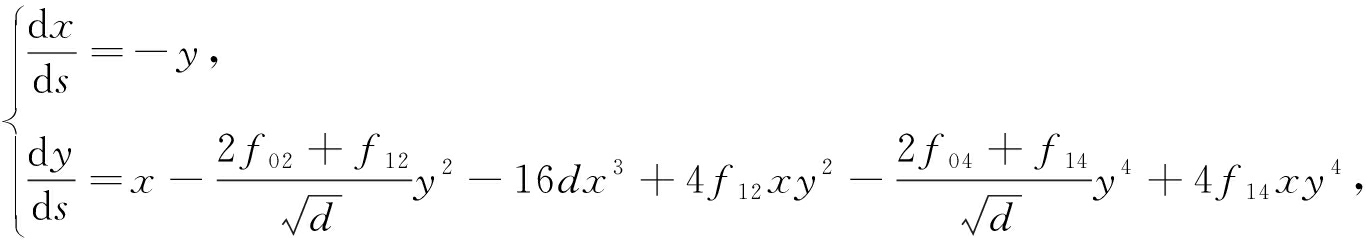(14)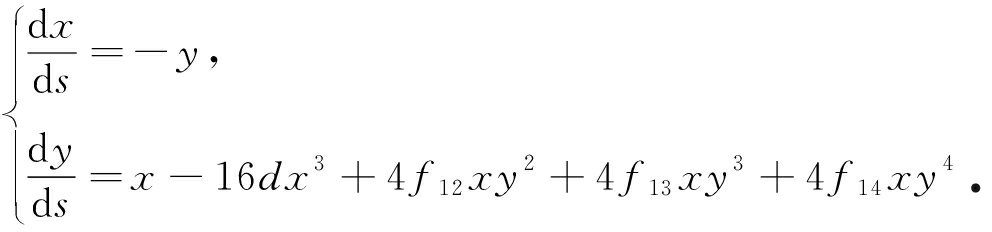(15)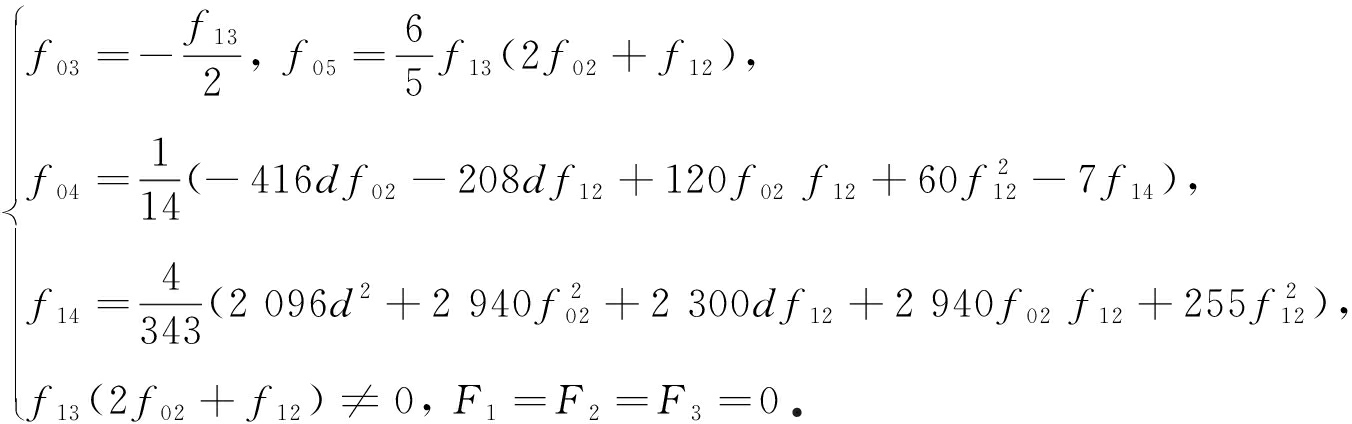(16)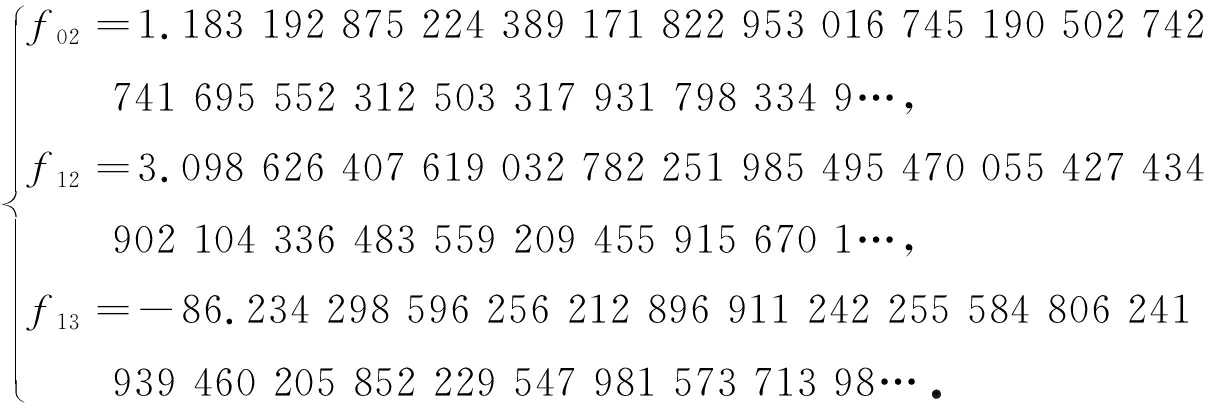(17)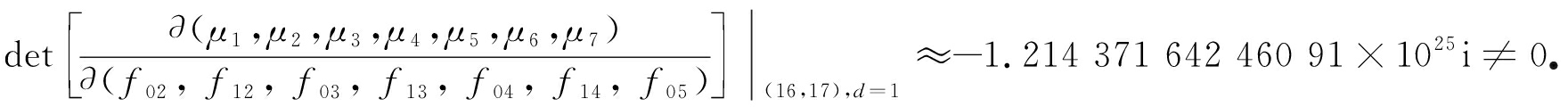## 3 细中心和局部临界周期分支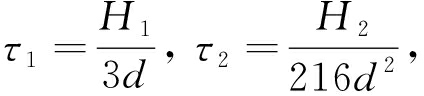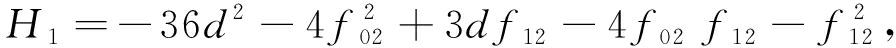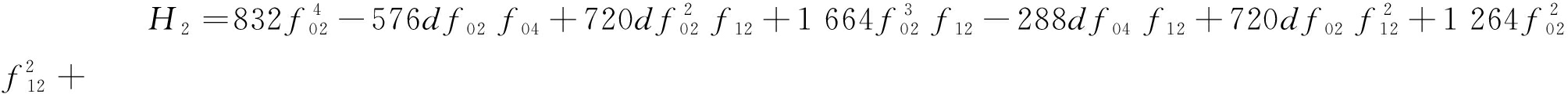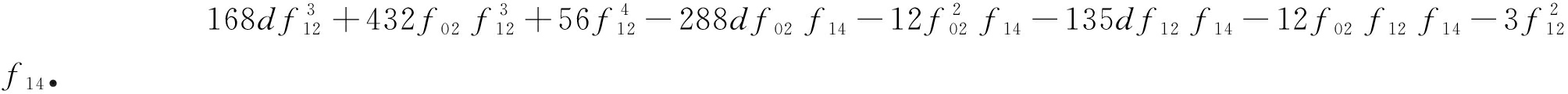f02=-f12/2，我们有τ1=f12-12d，令f12=12d，得τ1=0且τ2=(f14-192d2)/2．取f14=192d2，得τ2=0且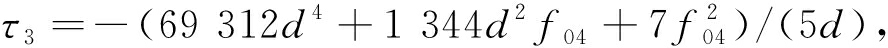不失一般性，我们假设d=1，容易发现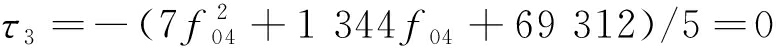没有实数解， 故对d>0， 有τ1=τ2=0, τ3≠0．因此， 系统(13)的原点是至多2阶的细中心．若f02≠-f12/2，令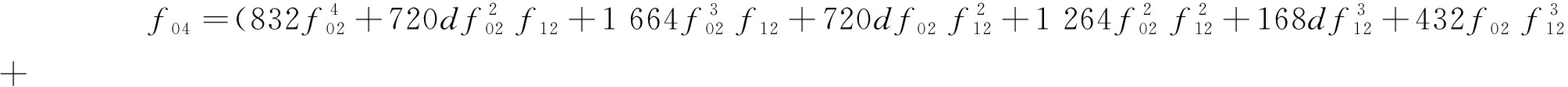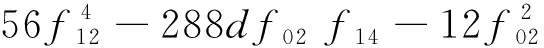f14-135df12 f14-12f02 f12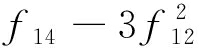f14)/(288d(2f02+f12)),

(18)

τ2=0．如果f14=0，我们有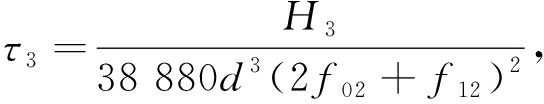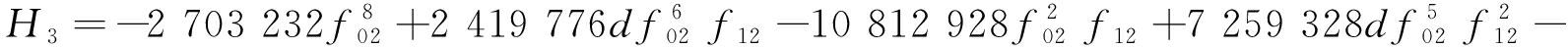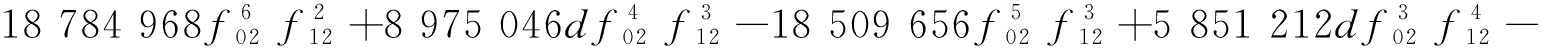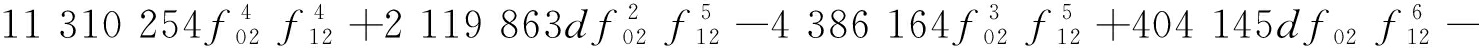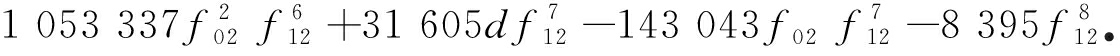G1=Res(H1,H3, f02)=

20 615 156 961 164 666 018 463 744-9 642 662 634 659 388 504 145 920 f12+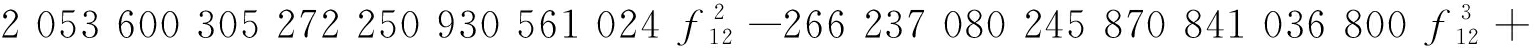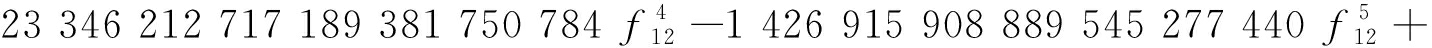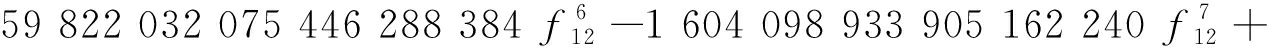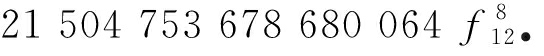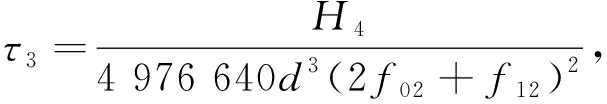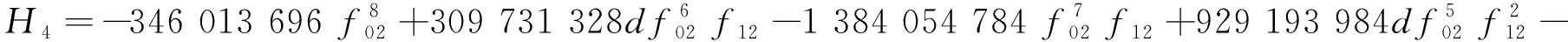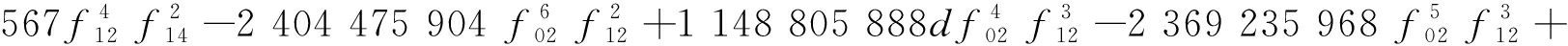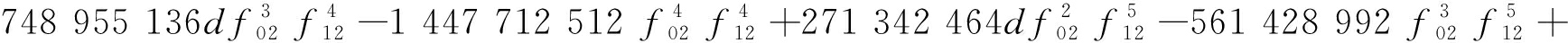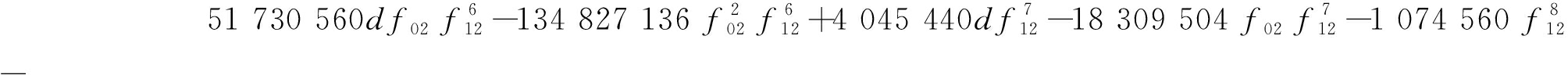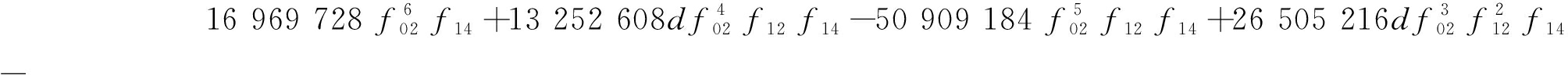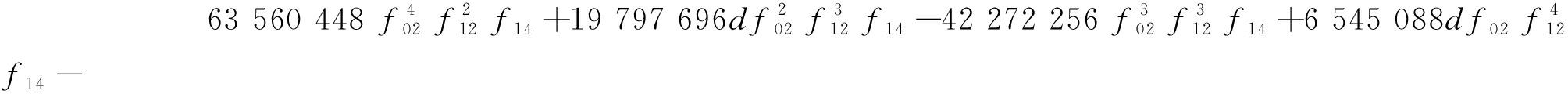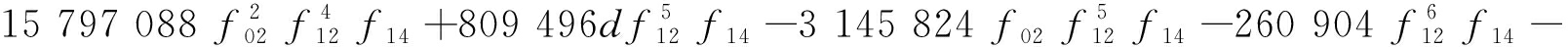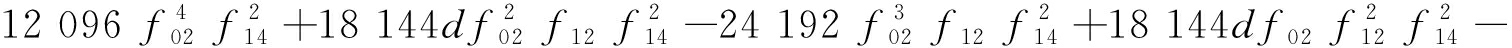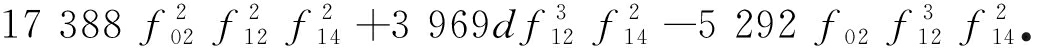G2=Res(H1,H4, f02)=

334 087 967 779 619 575 729 383 014 400-156 526 967 543 031 197 868 648 038 400 f12+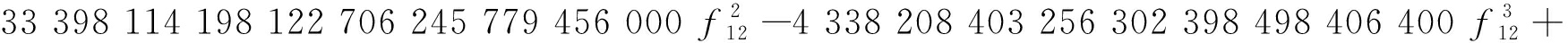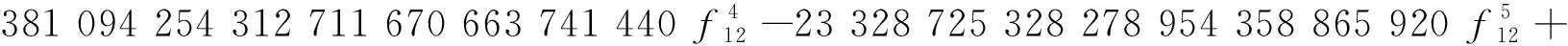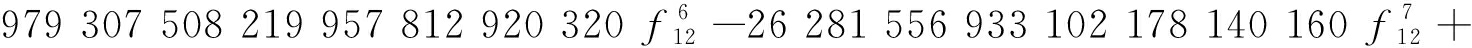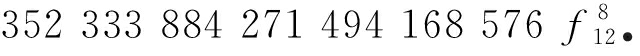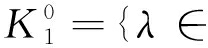R9: f03=f05=f13=0,H1≠0}，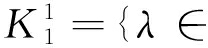R9: f03=f05=f13=0,H1=0,H2≠0}，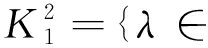R9: f03=f05=f13=0,H1=0,H2=0}.

P2=(12d-f12)π，P4=(192d2-f14)π/2， P6=(69 312d4+1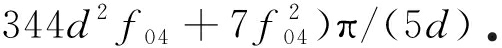λ*=(d, f01, f02, f12, f03, f13, f04, f14, f05)=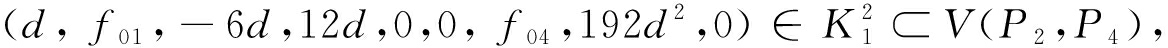λ*的任一邻域U，存在λ1=(d, f01,-6d,12d,0,0, f04,192d2+ε1,0)∈UV(P2)，这里ε1>0充分小，使得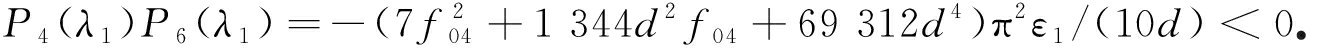再取

λ2=(d, f01,-6d,12d,0,0, f04,192d2-ε2,0)∈V(P2), P4(λ2)≠0,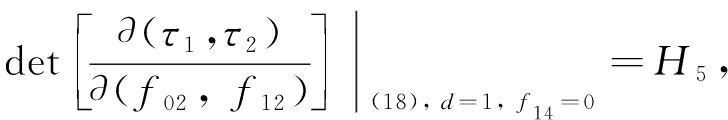(19)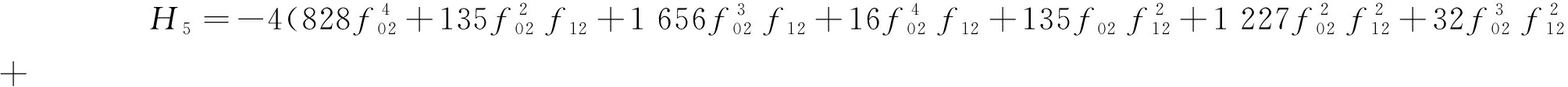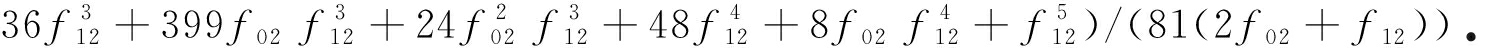G3=Res(H1,H5, f12)=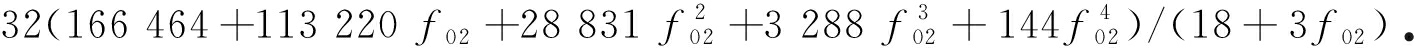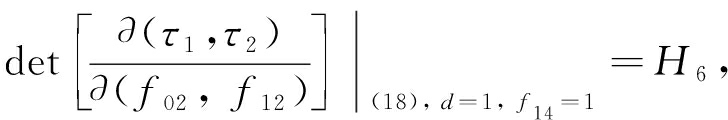(20)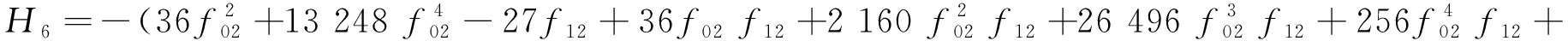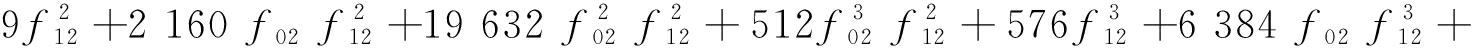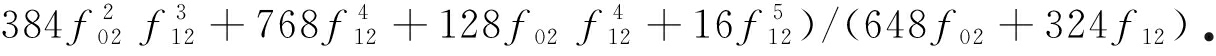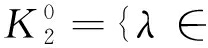R9: 2f03+f13=0, f05=0, 2f04+f14=0, f12=-2f02≠12d}，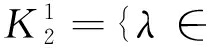R9: 2f03+f13=0, f05=0, f14=-2f04≠192d2, f12=-2f02=12d}，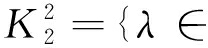R9: f13=-2f03,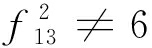400d3, f05=0, f14=-2f04=192d2, f12=-2f02=12d}，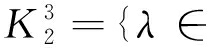R9: f13=-2f03,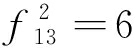400d3, f05=0, f14=-2f04=192d2, f12=-2f02=12d}.

λ*=(d, f01, f02, f12, f03, f13, f04, f14, f05)=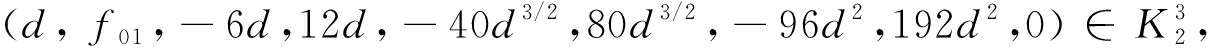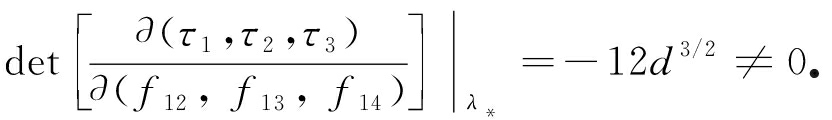## 4 结论和展望

 ARANSON I, KRAMER L. The world of the complex Ginzburg-Landau equation[J]. Review of Modern Physics, 2001, 74(1): 99-143.

 CHEN A Y, GUO L N, DENG X J. Existence of solitary waves and periodic waves for a perturbed generalized BBM equation[J]. Journal of Differential Equations, 2016, 261(10): 5324-5349.

 ZHUANG K G, DU Z J, LIN X J. Solitary waves solutions of singularly perturbed higher-order KdV equation via geometric singular perturbation method[J]. Nonlinear Dynamics, 2015, 80(1/2): 629-635.

 MANSOUR M B A. Traveling wave solutions of a reaction-diffusion model for bacterial growth[J]. Physica A: Statistical Mechanics and Its Applications, 2007, 383(2): 466-472.

 SHERRATT J A, SMITH M J. Periodic travelling waves in cyclic populations: field studies and reaction-diffusion models[J]. Journal of the Royal Society Interface, 2008, 5(22): 483-505.

 LI J B, WU J H, ZHU H P. Traveling waves for an integrable higher order KdV type wave equations[J]. International Journal of Bifurcation and Chaos, 2006, 16(8): 2235-2260.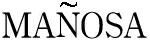V. Periodic travelling waves in nonlinear reaction-diffusion equations via multiple Hopf bifurcation[J]. Chaos, Solitons and Fractals, 2003, 18(2): 241-257.

 HUANG W T, CHEN T, LI J B. Isolated periodic wave trains and local critical wave lengths for a nonlinear reaction-diffusion equation[J]. Communications in Nonlinear Science and Numerical Simulation, 2019, 74(5): 84-96.

 SANCHEAGARDUNO F, MAINI P K. Traveling wave phenomena in some degenerate reaction-diffusion equations[J]. Journal of Differential Equations, 1995, 117(2): 281-319.

 YANG G X. Hopf bifurcation of traveling wave solutions of delayed Fisher-KPP equation[J]. Applied Mathematics and Computation, 2013, 220(4): 213-220.

 CHICONE C, JACOBS M. Bifurcation of critical periods for plane vector fields[J]. Transactions of the American Mathematical Society, 1989, 312(2): 433-486.

 ROMANOVSKI V G, HAN M A. Critical period bifurcations of a cubic system[J]. Journal of Physics A: Mathematical and General, 2003, 36(18): 5011-5022.

 ROUSSEAU C, TONI B. Local bifurcations of critical periods in the reduced Kukles system[J]. Canadian Journal of Mathematics, 1997, 49(2): 338-358.

 YU P, HAN M A. Critical periods of planar revertible vector field with third-degree polynomial functions[J]. International Journal of Bifurcation and Chaos, 2009, 19(1): 419-433.

 LIU Y R, LI J B. Theory of values of singular point in complex autonomous differential systems[J]. Science in China (Series A), 1990, 33: 10-24.

 黄文韬. 微分自治系统的几类极限环分支与等时中心问题[D]. 博士学位论文. 长沙: 中南大学, 2004. (HUANG Wentao. Several classes of bifurcations of limit cycles and isochronous centers for differential autonomous systems[D]. PhD Thesis. Changsha: Central South University, 2004. (in Chinese))

 LIU Y R, HUANG W T. A new method to determine isochronous center conditions for polynomial differential systems[J]. Bulletin des Sciences Mathématiques, 2003, 127(2): 133-148.

 YU P, HAN M A. Twelve limit cycles in a cubic case of the 16th Hilbert problem[J]. International Journal of Bifurcation and Chaos, 2005, 15(7): 2191-2205.

 CHEN H B, LIU Y R. Linear recursion formulas of quantities of singular point and applications[J]. Applied Mathematics and Computation, 2004, 148(1): 163-171.

 GEYER A, VILLADELPRAT J. On the wave length of smooth periodic traveling waves of the Camassa-Holm equation[J]. Journal of Differential Equations, 2015, 259(6): 2317-2332.

# Solitary Periodic Waves and Local Bifurcations of Critical Periods for a Class of Reaction-Diffusion Equations

GU Jieping1, HUANG Wentao1, CHEN Ting2

(1. College of Mathematics and Statistics, Guangxi Normal University,Guilin, Guangxi 541006, P.R.China;2. School of Statistics and Mathematics, Guangdong University of Finance and Economics,Guangzhou 510320, P.R.China)

Abstract: The small-amplitude solitary periodic wave solutions and the local critical periodic bifurcations of the traveling wave equations for a class of reaction-diffusion equations with quintic nonlinear reaction terms and constant diffusion terms were studied. First, the reaction-diffusion equation was transformed into the corresponding traveling wave system through traveling wave transformation. The first 8 singular point quantities of the system were calculated with the singular point value method and the computer algebra software MATHEMATICA. Then, 2 center conditions for the singular point of the system were obtained, 8 limit cycles were proved to bifurcate at the origin of the traveling wave system, and 8 small-amplitude solitary periodic wave solutions were found to exist in the corresponding nonlinear reaction-diffusion equation. Furthermore, through computation of the period constants, the weak center order for the origin of the traveling wave system was derived. Then, the system was proved to have at most 3 local critical periodic bifurcations and be able to reach the 3 bifurcations. Moreover, the analysis of the critical periodic bifurcations of the traveling wave system reveals that the reaction-diffusion equation has 3 critical periodic wavelengths.

Key words: reaction-diffusion equation; singular point quantity; limit cycle; solitary periodic wave; critical period bifurcation

DOI：10.21656/1000-0887.410263

*收稿日期： 2020-09-07； 修订日期：2020-09-23